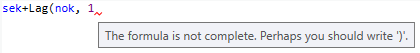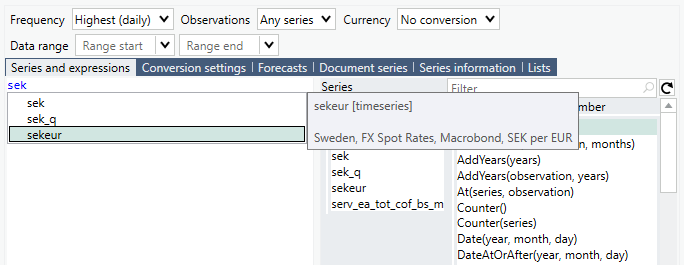# Overview

The purpose of the Macrobond formula language is to make calculations on series. The series are typically time series and the result of a formula is a time series. By using formulas you can combine arithmetic operations with built in functions to create powerful calculations. You can find a complete list of built-in functions here.

## Important information

Formulas can be utilized in both the Series list and Formula analysis. The method of writing formulas is identical between the two except for how references to series are written. The instructions below are targeted at making calculations in the Series list. Thus when referencing to a series, the name of the series will be used and not an alias. To learn more about aliases and the differences between using the Series list and the Formula analysis for calculations, click here.

# How calculation works

## Series names

You can use the name of a series from the database in formulas. A very simple example is:

sek

Which references to the Macrobond series called sek.

## Numerical constants

Examples of numerical constants are:

123

-123

123.456

123.456e-3

## Arithmetic operations

An example of a simple formula is the following:

 sek/nok

which calculates the cross rate between the Swedish and the Norwegian krona.

The time series sek and nok are treated as vectors of values. In a vector, each value can either be a number or a null value.

Before any calculations are made, all series in the formulas are converted to the same frequency. The series also need to be the same length. This is achieved by adding null values to the beginning of the vectors until they are of equal length.

### Example

The division operator / takes two series and produces a new series by dividing the corresponding values in the two series. For most calculations, the result is null when any of the values is null.

This can be illustrated by the use of a fictional example:

 Observation number sek nok sek/nok 1971-01-04 0 5.1643 1971-01-05 1 5.1628 1971-01-06 2 7.1367 5.1614 0.723219 1971-01-07 3 7.1386 5.1649 0.723517 1971-01-08 4 7.1382 5.1631 0.723306 1971-01-11 5 7.1390 5.1642 0.723379

The operators, such as +, - and * handles null in the same way.

## Functions

In addition to the arithmetic operators there are also functions. A function can take zero or more parameters. Parameters are written within parentheses and separated with commas.

### Example

An example of a formula using a function is the following:

 Sum(sek)

This will calculate the sum of all values in the series. With the same fictional values, the result would look like this:

 Observation number sek Sum(sek) 1971-01-06 0 7.1367 42.8326 1971-01-07 1 7.1386 42.8326 1971-01-08 2 7.1382 42.8326 1971-01-11 3 7.1390 42.8326 1971-01-12 4 7.1411 42.8326 1971-01-13 5 7.1390 42.8326

Another example is the following formula:

 sek*100

The result is a new series where each value is multiplied by 100. There is no function multiplying a series with a constant. Instead, the formula interpreter will create a series where each value is 100 and then multiply these series:

 Observation number sek constant sek*100 1971-01-06 0 7.1367 100 713.67 1971-01-07 1 7.1386 100 713.86 1971-01-08 2 7.1382 100 713.82 1971-01-11 3 7.1390 100 713.90 1971-01-12 4 7.1411 100 714.11 1971-01-13 5 7.1390 100 713.90

In most cases you do not need to know about the conversion of constants to series, but it can help you to understand what happens with a formula like this:

Sum(100)

If you have this formula together with the fictional series sek, the result would look like this:

 Observation number sek Sum(100) 1971-01-06 0 7.1367 600 1971-01-07 1 7.1386 600 1971-01-08 2 7.1382 600 1971-01-11 3 7.1390 600 1971-01-12 4 7.1411 600 1971-01-13 5 7.1390 600

You get the value 600 because the constant 100 is converted to a series with six values of 100 and then this series is summed.

### Observation windows (rolling)

There are functions in Macrobond with the same name that takes different number of parameters. Many of these functions takes a parameter that specifies the length of a window. With them you can create rolling series.

### Example

 Sum(sek) This is the sum of all values in the series. Sum(sek, 3) This is the sum of three observations including the current observation and the two previous ones.

If there are not enough data points to fill the window, the values will be null.  This is depicted in the table below.

 Observation number sek Sum(sek,3) 1971-01-06 0 7.1367 1971-01-07 1 7.1386 1971-01-08 2 7.1382 21.4135 1971-01-11 3 7.1390 21.4158 1971-01-12 4 7.1411 21.4183 1971-01-13 5 7.1390 21.4191

Another useful example of a function that takes a window length is Mean(series, window). The result of this function is also known as the moving average.

### Observation numbers

Each position in the series has an ordinal number called an observation number. You can get a series of the ordinal numbers with the function Counter().

 Observation number sek Counter() 1971-01-06 0 7.1367 0 1971-01-07 1 7.1386 1 1971-01-08 2 7.1382 2 1971-01-11 3 7.1390 3 1971-01-12 4 7.1411 4 1971-01-13 5 7.1390 5

If it is a time series, each ordinal number is associated with a date.

Please note that you should never make any assumption about what the ordinal numbers are. Thus, you should not use an expression like At(sek, 0) to get the first value of a series.The ordinal number of the first observation can be any number. Instead you should use FirstValid(sek), which returns the ordinal number of the first observation that is not null.

### Example

There are a number of functions related to dates and ordinal numbers. A useful function is Date(year, month, day) that returns the ordinal number for a specific date.

 Observation number sek Date(1971,1,8) 1971-01-06 0 7.1367 2 1971-01-07 1 7.1386 2 1971-01-08 2 7.1382 2 1971-01-11 3 7.1390 2 1971-01-12 4 7.1411 2 1971-01-13 5 7.1390 2

With the function At(series, observation) you can get the value at a specific observation number.

 Observation number sek At(sek, Date(1971,1,8)) 1971-01-06 0 7.1367 7.1382 1971-01-07 1 7.1386 7.1382 1971-01-08 2 7.1382 7.1382 1971-01-11 3 7.1390 7.1382 1971-01-12 4 7.1411 7.1382 1971-01-13 5 7.1390 7.1382

### Logical values and functions

The logical values in a series are represented by 1 for True and 0 for False.

### Example

In the following example the operator > (“greater than”) is used to compare a series with a lagged series. The result will be True (1) when the value has increased since the last observation.

 Observation number sek Lag(sek, 1) sek > Lag(sek, 1) 1971-01-06 0 7.1367 1971-01-07 1 7.1386 7.1367 1 1971-01-08 2 7.1382 7.1386 0 1971-01-11 3 7.1390 7.1382 1 1971-01-12 4 7.1411 7.1390 1 1971-01-13 5 7.1390 7.1411 0 1971-01-14 6 7.1390

The function If is commonly used with logical expressions. The function takes three parameters. The first parameter is interpreted as a logical expression that determines if the result should be the value of the second or the third parameter.

The following example replaces any null values (missing values) with the highest value of the last ten observations
If (IsNull(sek),Hi(sek, 10), sek)

You can write comments in formulas by typing two slashes “//”. Everything after the slashes to the end of the line, will be regarded as a comment and not part of the formula expression.

## Operators

All operators work on constants and series. Here are the operators sorted in order of precedence. Parentheses can be used to further control the order of evaluation.

 Operator Description Precedence - Unary minus 7 * Multiplication 6 / Division 6 + Plus 5 - Minus 5 ! Logical “not” 4 > Greater than 3 >= Greater than or equal 3 < Less than 3 <= Less than or equal 3 = Equal 3 <> Not equal 3 & Logical “and” 2 | Logical “or” 1

# The formula editor

The Formula editor helps you write formulas. In the Series list, you can bring up the Formula editor by clicking on the fx button to the right of an expression, or by pressing Ctrl+E on the keyboard.

Some of the useful features of the editor are:

• You can double click on a function in the list to the right in order to insert a function.
• You can filter the list of functions by typing a text in the box above the list of functions. Only functions that contain the text in the function name or description will be included in the list.
• The tooltip of a function in the list or in an expression will show details about the function:• The tooltip of a series will show the title of the series:• When the text cursor is just before a left parenthesis or directly after a right parenthesis , a blue shade on the background will show the area between the matching parentheses:• When the text cursor is within or next to an identifier, all instances of that identifier in the expression are shaded:• The editor will check the syntax as you write and show a red squiggle if there is an error. You can see the error text at the bottom of the editor or as a tooltip:• Numerical constants are dark red. Series, parameters and variables are blue. Comments are green.
• The series used in the document will be displayed at the end of list of functionsThe tooltip will tell you the title of the series. You can double click to enter them into the formula.• Auto-suggestions for series name. When you start typing a series name in the Formula editor, suggestions will be shown if the text you type matches the start of a series in the document or any of the series you have marked as favorite. Use the mouse or press the down arrow to walk the list. The tooltip will give you the series title. Press ‘Enter’ to select.# User defined functions

User defined functions are a way to structure formula expressions and to reuse commonly used expressions. It enables you to both reduce the amount of formulas you write and to save functions for future use. For more information see: User defined formulas